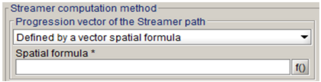# Streamer Criterion: Computing method

## Introduction

This section concerns the tool's second tab called “computation method”.

## Progression vector of the Streamer path

As said previously, the Streamer paths are approximated by physical quantities evolution laws: in Flux, the electric field lines are considered by default.
Note: The opposite electric field -E may be selected, in case of selecting a line (Flux 2D) or a face (Flux 3D) of a ground region (0V) as a starting point, the Streamer criterion cannot be computed.

It is also possible to choose another progression vector, defined by a vector spatial formula.## Streamer path discretization

The path discretization is important to obtain a precise integration result.

Three discretization methods exist, each one defines a specific discretization step. Then, the Euler-Cauchy method is applied to divide this discretization step n times (n is the “Number of Euler-Cauchy substeps” chosen by user).

The three discretization methods are presented below:

• Fixed : the discretization path is defined by a fixed value
• Adaptive with mesh size elements: the discretization path follows the corresponding mesh element size. This method is advised because it has the best ratio “results precision / computation time”.
• Adaptive by angle minimization: it corresponds to an improvement of the previous method. The initial discretization step is chosen in the same way as the previous method, and it is subdivided until the angle between two consecutive electric field arrows becomes lower than 1°. This method can be more precise than the others in some cases (if the electric field lines are curved), but the computation time can increase.

## Integration mode

Actually, a Streamer won't go necessarily from the high potential conductor.

Given that Flux imposes a path starting from the high potential conductor, this option can remedy this problem.

It has an impact when the electric field E is not homogeneous along the path.

In fact, if E decreases until αeff becomes negative, there are two possibilities for the integral computing:

• "Integrate starting only from the conductor” means that αeff will be systematically integrated on the orange part of the figure (and never on the green part)
• “Integrate from the both sides of the path” means that the maximum between αeff integral (K) value on the orange and the green part will be taken into account. The integration giving the maximum value indicates the Streamer starting side. A graphical arrow will indicate the streamer propagation direction.## Computation mode

This option allows to choose:

• A parallelized computation
• A sequential computation
• An automatic computation: it corresponds to the parallelized computation in most of the time, except some cases which can not use the parallelized computation because of code limitation (the sequential computation is used).

## Dichotomy

The dichotomy parameters are defined by:

• The maximum number of iterations
• The relative accuracy on K